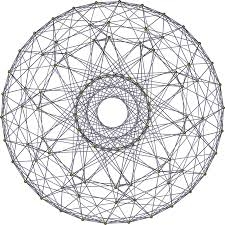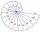# Cube root

Find cube root of 18

x =  2.6207

### Step-by-step explanation:Did you find an error or inaccuracy? Feel free to write us. Thank you!## Related math problems and questions:

• PoolIf water flows into the pool by two inlets, fill the whole for 19 hours. The first inlet filled pool 5 hour longer than the second. How long pool take to fill with two inlets separately?
• The volume 2The volume of a cube is 27 cubic meters. Find the height of the cube.
• The ticketsThe tickets to the show cost some integer number greater than 1. Also, the sum of the price of the children's and adult tickets, as well as their product, was the power of the prime number. Find all possible ticket prices.
• RotationThe right triangle with legs 11 cm and 18 cm rotate around the longer leg. Calculate the volume and surface area of the formed cone.
• Triangle ABCCalculate the sides of triangle ABC with area 1404 cm2 and if a: b: c = 12:7:18
• RootUse law of square roots roots: ?
• Cube zoomHow many percents do we increase the volume and surface of the cube if we magnify its edge by 38 %?
• An exampleAn example is playfully for grade 6 from Math and I don't know how to explain it to my daughter when I don't want to use the calculator to calculate the cube root. Thus: A cuboid was made from a block of 16x18x48 mm of modeline. What will be the edge of t
• Cube in a sphereThe cube is inscribed in a sphere with a volume 7253 cm3. Determine the length of the edges of a cube.
• The sum 13The sum of the exponents of the prime factors in the prime factorization of 196 is. .. .
• PowerNumber ?. Find the value of x.
• HoleIn the center of the cube with edge 14 cm we will drill cylinder shape hole. Volume of the hole must be 27% of the cube. What drill diameter should be chosen?
• CubesOne cube is an inscribed sphere and the other one described. Calculate the difference of volumes of cubes, if the difference of surfaces in 257 mm2.
• CylindersThe area of the side of two cylinders is the same rectangle of 33 mm × 18 mm. Which cylinder has a larger volume and by how much?
• Cube surface and volumeThe surface of the cube is 500 cm2, how much cm3 will be its volume?
• Area of a cubeCalculate the surface area of a cube if its volume is equal to 729 cubic meters.
• Big cubeCalculate the surface of the cube, which is composed of 64 small cubes with an edge 1 cm long.